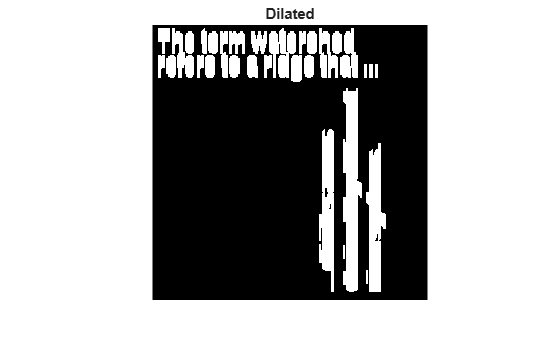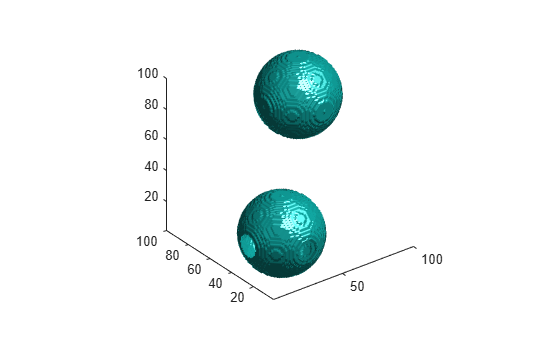Documentation

# imdilate

## Syntax

``J = imdilate(I,SE)``
``J = imdilate(I,nhood)``
``J = imdilate(___,packopt)``
``J = imdilate(___,shape)``

## Description

example

````J = imdilate(I,SE)` dilates the grayscale, binary, or packed binary image `I`, returning the dilated image, `J`. `SE` is a structuring element object or array of structuring element objects, returned by the `strel` or `offsetstrel` functions.You optionally can perform the dilation using a GPU (requires Parallel Computing Toolbox™).```
````J = imdilate(I,nhood)` dilates the image `I`, where `nhood` is a matrix of `0`s and `1`s that specifies the structuring element neighborhood. `imdilate` determines the center element of the neighborhood by `floor((size(nhood)+1)/2)`.This syntax is equivalent to `imdilate(I,strel(nhood))`.```
````J = imdilate(___,packopt)` specifies whether `I` is a packed binary image.This syntax is not supported on a GPU.```

example

````J = imdilate(___,shape)` specifies the size of the output image.```

## Examples

collapse all

Read a binary image into the workspace.

`BW = imread('text.png');`

Create a vertical line shaped structuring element.

`se = strel('line',11,90);`

Dilate the image with a vertical line structuring element and compare the results.

```BW2 = imdilate(BW,se); imshow(BW), title('Original')````figure, imshow(BW2), title('Dilated')`Read a grayscale image into the workspace.

`originalI = imread('cameraman.tif');`

Create a nonflat ball-shaped structuring element.

`se = offsetstrel('ball',5,5);`

Dilate the image.

`dilatedI = imdilate(originalI,se);`

Display the original image and the dilated image.

`imshowpair(originalI,dilatedI,'montage')`Create two flat, line-shaped structuring elements, one at 0 degrees and the other at 90 degrees.

`se1 = strel('line',3,0)`
```se1 = strel is a line shaped structuring element with properties: Neighborhood: [1 1 1] Dimensionality: 2 ```
`se2 = strel('line',3,90)`
```se2 = strel is a line shaped structuring element with properties: Neighborhood: [3x1 logical] Dimensionality: 2 ```

Dilate the scalar value 1 with both structuring elements in sequence, using the `'full'` option.

`composition = imdilate(1,[se1 se2],'full')`
```composition = 3×3 1 1 1 1 1 1 1 1 1 ```

Create a logical 3D volume with two points.

```BW = false(100,100,100); BW(25,25,25) = true; BW(75,75,75) = true;```

Dilate the 3D volume using a spherical structuring element.

```se = strel('sphere',25); dilatedBW = imdilate(BW,se);```

Visualize the dilated image volume.

```figure isosurface(dilatedBW, 0.5)```## Input Arguments

collapse all

Input image, specified as a grayscale image, binary image, or packed binary image of any dimension.

Data Types: `single` | `double` | `int8` | `int16` | `int32` | `uint8` | `uint16` | `uint32` | `logical`

Structuring element, specified as a scalar `strel` object or `offsetstrel` object. `SE` can also be an array of `strel` object or `offsetstrel` objects, in which case `imdilate` performs multiple dilations of the input image, using each structuring element in succession.

`imdilate` performs grayscale dilation for all images except images of data type `logical`. In this case, the structuring element must be flat and `imdilate` performs binary dilation.

Structuring element neighborhood, specified as a matrix of `0`s and `1`s.

Example: `[0 1 0; 1 1 1; 0 1 0]`

Indicator of packed binary image, specified as one of the following.

Value

Description

`'notpacked'`

`I` is treated as a normal array.

`'ispacked'`

`I` is treated as a packed binary image as produced by `bwpack`. `I` must be a 2-D `uint32` array and `SE` must be a flat 2-D structuring element. The value of `shape` must be `'same'`.

Data Types: `char` | `string`

Size of the output image, specified as one of the following.

Value

Description

`'same'`

The output image is the same size as the input image. If the value of `packopt` is `'ispacked'`, then `shape` must be `'same'`.

`'full'`

Compute the full dilation.

Data Types: `char` | `string`

## Output Arguments

collapse all

Dilated image, returned as a grayscale image, binary image, or packed binary image. If the input image `I` is packed binary, then `J` is also packed binary. `J` has the same class as `I`.

collapse all

### Binary Dilation

The binary dilation of A by B, denoted AB, is defined as the set operation:

`$A\oplus B=\left\{z|{\left(\stackrel{^}{B}\right)}_{z}\cap A\ne \varnothing \right\},$`

where $\stackrel{^}{B}$ is the reflection of the structuring element B. In other words, it is the set of pixel locations z, where the reflected structuring element overlaps with foreground pixels in A when translated to z. Note that some applications use a definition of dilation in which the structuring element is not reflected.

### Grayscale Dilation

In the general form of grayscale dilation, the structuring element has a height. The grayscale dilation of A(x, y) by B(x, y) is defined as:

`$\left(A\oplus B\right)\left(x,y\right)=\mathrm{max}\left\{A\left(x-{x}^{\prime },y-{y}^{\prime }\right)+B\left({x}^{\prime },{y}^{\prime }\right)|\left({x}^{\prime },{y}^{\prime }\right)\in {D}_{B}\right\},$`

where DB is the domain of the structuring element B and A(x, y) is assumed to be –∞ outside the domain of the image. To create a structuring element with nonzero height values, use the syntax `strel(nhood,height)`, where `height` gives the height values and `nhood` corresponds to the structuring element domain, DB.

Most commonly, grayscale dilation is performed with a flat structuring element (B(x,y) = 0). Grayscale dilation using such a structuring element is equivalent to a local-maximum operator:

`$\left(A\oplus B\right)\left(x,y\right)=\mathrm{max}\left\{A\left(x-{x}^{\prime },y-{y}^{\prime }\right)|\left({x}^{\prime },{y}^{\prime }\right)\in {D}_{B}\right\}.$`

All of the `strel` syntaxes except for `strel(nhood,height)`, `strel('arbitrary',nhood,height)`, and `strel('ball',...)` produce flat structuring elements.

## Algorithms

`imdilate` automatically takes advantage of the decomposition of a structuring element object (if it exists). Also, when performing binary dilation with a structuring element object that has a decomposition, `imdilate` automatically uses binary image packing to speed up the dilation.

Dilation using bit packing is described in .

 Gonzalez, R. C., R. E. Woods, and S. L. Eddins, Digital Image Processing Using MATLAB, Gatesmark Publishing, 2009.

 Haralick, R. M., and L. G. Shapiro, Computer and Robot Vision, Vol. I, Addison-Wesley, 1992, pp. 158-205.

 van den Boomgard, R, and R. van Balen, "Methods for Fast Morphological Image Transforms Using Bitmapped Images," Computer Vision, Graphics, and Image Processing: Graphical Models and Image Processing, Vol. 54, Number 3, pp. 254-258, May 1992.